# gamultiobj

Find Pareto front of multiple fitness functions using genetic algorithm

## Syntax

``x = gamultiobj(fun,nvars)``
``x = gamultiobj(fun,nvars,A,b)``
``x = gamultiobj(fun,nvars,A,b,Aeq,beq)``
``x = gamultiobj(fun,nvars,A,b,Aeq,beq,lb,ub)``
``x = gamultiobj(fun,nvars,A,b,Aeq,beq,lb,ub,nonlcon)``
``x = gamultiobj(fun,nvars,A,b,Aeq,beq,lb,ub,options)``
``````x = gamultiobj(fun,nvars,A,b,Aeq,beq,lb,ub,nonlcon,options)``````
``x = gamultiobj(fun,nvars,A,b,Aeq,beq,lb,ub,nonlcon,intcon)``
``````x = gamultiobj(fun,nvars,A,b,Aeq,beq,lb,ub,nonlcon,intcon,options)``````
``x = gamultiobj(problem)``
``````[x,fval] = gamultiobj(___)``````
``````[x,fval,exitflag,output] = gamultiobj(___)``````
``````[x,fval,exitflag,output,population,scores] = gamultiobj(___)``````

## Description

example

````x = gamultiobj(fun,nvars)` finds `x` on the Pareto Front of the objective functions defined in `fun`. `nvars` is the dimension of the optimization problem (number of decision variables). The solution `x` is local, which means it might not be on the global Pareto front. NotePassing Extra Parameters explains how to pass extra parameters to the objective function and nonlinear constraint functions, if necessary. ```

example

````x = gamultiobj(fun,nvars,A,b)` finds a local Pareto set `x` subject to the linear inequalities $A\ast x\le b$. See Linear Inequality Constraints. `gamultiobj` supports linear constraints only for the default `PopulationType` option (`'doubleVector'`).```
````x = gamultiobj(fun,nvars,A,b,Aeq,beq)` finds a local Pareto set `x` subject to the linear equalities $Aeq\ast x=beq$ and the linear inequalities $A\ast x\le b$, see Linear Equality Constraints. (Set `A = []` and `b = []` if no inequalities exist.) `gamultiobj` supports linear constraints only for the default `PopulationType` option (`'doubleVector'`).```

example

````x = gamultiobj(fun,nvars,A,b,Aeq,beq,lb,ub)` defines a set of lower and upper bounds on the design variables `x` so that a local Pareto set is found in the range `lb `≤` x `≤` ub`, see Bound Constraints. Use empty matrices for `Aeq` and `beq` if no linear equality constraints exist. `gamultiobj` supports bound constraints only for the default `PopulationType` option (`'doubleVector'`).```
````x = gamultiobj(fun,nvars,A,b,Aeq,beq,lb,ub,nonlcon)` finds a Pareto set subject to the constraints defined in `nonlcon`. The function `nonlcon` accepts `x` and returns vectors `c` and `ceq`, representing the nonlinear inequalities and equalities respectively. `gamultiobj` minimizes `fun` such that `c(x) `≤` 0` and `ceq(x) = 0`. (Set `lb = []` and `ub = []` if no bounds exist.) `gamultiobj` supports nonlinear constraints only for the default `PopulationType` option (`'doubleVector'`).```

example

````x = gamultiobj(fun,nvars,A,b,Aeq,beq,lb,ub,options)` or ```x = gamultiobj(fun,nvars,A,b,Aeq,beq,lb,ub,nonlcon,options)``` finds a Pareto set `x` with the default optimization parameters replaced by values in `options`. Create `options` using `optimoptions` (recommended) or a structure.```

example

````x = gamultiobj(fun,nvars,A,b,Aeq,beq,lb,ub,nonlcon,intcon)` or ```x = gamultiobj(fun,nvars,A,b,Aeq,beq,lb,ub,nonlcon,intcon,options)``` requires that the variables listed in `intcon` take integer values. NoteWhen there are integer constraints, `gamultiobj` does not accept nonlinear equality constraints, only nonlinear inequality constraints. ```
````x = gamultiobj(problem)` finds the Pareto set for `problem`, where `problem` is a structure described in `problem`.```
``````[x,fval] = gamultiobj(___)```, for any input variables, returns a matrix `fval`, the value of all the fitness functions defined in `fun` for all the solutions in `x`. `fval` has `nf` columns, where `nf` is the number of objectives, and has the same number of rows as `x`.```
``````[x,fval,exitflag,output] = gamultiobj(___)``` returns `exitflag`, an integer identifying the reason the algorithm stopped, and `output`, a structure that contains information about the optimization process.```

example

``````[x,fval,exitflag,output,population,scores] = gamultiobj(___)``` returns `population`, whose rows are the final population, and `scores`, the scores of the final population.```

## Examples

collapse all

Find the Pareto front for a simple multiobjective problem. There are two objectives and two decision variables `x`.

`fitnessfcn = @(x)[norm(x)^2,0.5*norm(x(:)-[2;-1])^2+2];`

Find the Pareto front for this objective function.

```rng default % For reproducibility x = gamultiobj(fitnessfcn,2);```
```Optimization terminated: average change in the spread of Pareto solutions less than options.FunctionTolerance. ```

Plot the solution points.

```plot(x(:,1),x(:,2),'ko') xlabel('x(1)') ylabel('x(2)') title('Pareto Points in Parameter Space')```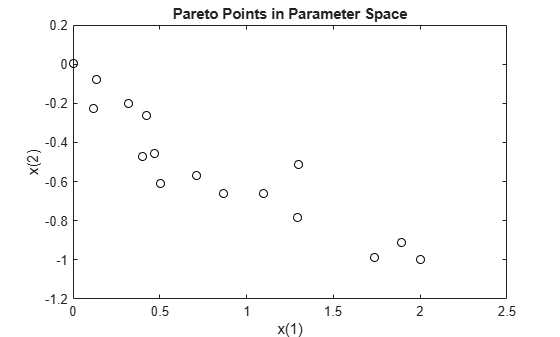To see the effect of a linear constraint on this problem, see Multiobjective Problem with Linear Constraint.

This example shows how to find the Pareto front for a multiobjective problem in the presence of a linear constraint.

There are two objective functions and two decision variables `x`.

`fitnessfcn = @(x)[norm(x)^2,0.5*norm(x(:)-[2;-1])^2+2];`

The linear constraint is $x\left(1\right)+x\left(2\right)\le 1/2$.

```A = [1,1]; b = 1/2;```

Find the Pareto front.

```rng default % For reproducibility x = gamultiobj(fitnessfcn,2,A,b);```
```Optimization terminated: average change in the spread of Pareto solutions less than options.FunctionTolerance. ```

Plot the constrained solution and the linear constraint.

```plot(x(:,1),x(:,2),'ko') t = linspace(-1/2,2); y = 1/2 - t; hold on plot(t,y,'b--') xlabel('x(1)') ylabel('x(2)') title('Pareto Points in Parameter Space') hold off```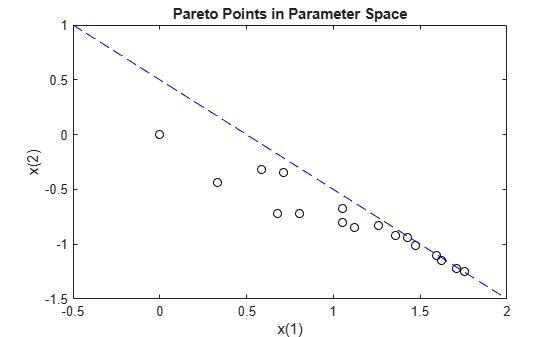To see the effect of removing the linear constraint from this problem, see Simple Multiobjective Problem.

Find the Pareto front for the two fitness functions `sin(x)` and `cos(x)` on the interval $0\le x\le 2\pi$.

```fitnessfcn = @(x)[sin(x),cos(x)]; nvars = 1; lb = 0; ub = 2*pi; rng default % for reproducibility x = gamultiobj(fitnessfcn,nvars,[],[],[],[],lb,ub)```
```Optimization terminated: average change in the spread of Pareto solutions less than options.FunctionTolerance. ```
```x = 18×1 4.7124 4.7124 3.1415 3.6733 3.9845 3.4582 3.9098 4.4409 4.0846 3.8686 ⋮ ```

Plot the solution. `gamultiobj` finds points along the entire Pareto front.

```plot(sin(x),cos(x),'r*') xlabel('sin(x)') ylabel('cos(x)') title('Pareto Front') legend('Pareto front')```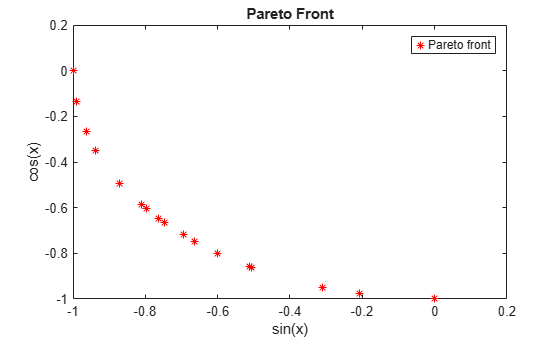Find and plot the Pareto front for the two-objective Schaffer's second function. This function has a disconnected Pareto front.

Copy this code to a function file on your MATLAB® path.

```function y = schaffer2(x) % y has two columns % Initialize y for two objectives and for all x y = zeros(length(x),2); % Evaluate first objective. % This objective is piecewise continuous. for i = 1:length(x) if x(i) <= 1 y(i,1) = -x(i); elseif x(i) <=3 y(i,1) = x(i) -2; elseif x(i) <=4 y(i,1) = 4 - x(i); else y(i,1) = x(i) - 4; end end % Evaluate second objective y(:,2) = (x -5).^2; ```

Plot the two objectives.

```x = -1:0.1:8; y = schaffer2(x); plot(x,y(:,1),'r',x,y(:,2),'b'); xlabel x ylabel 'schaffer2(x)' legend('Objective 1','Objective 2') ```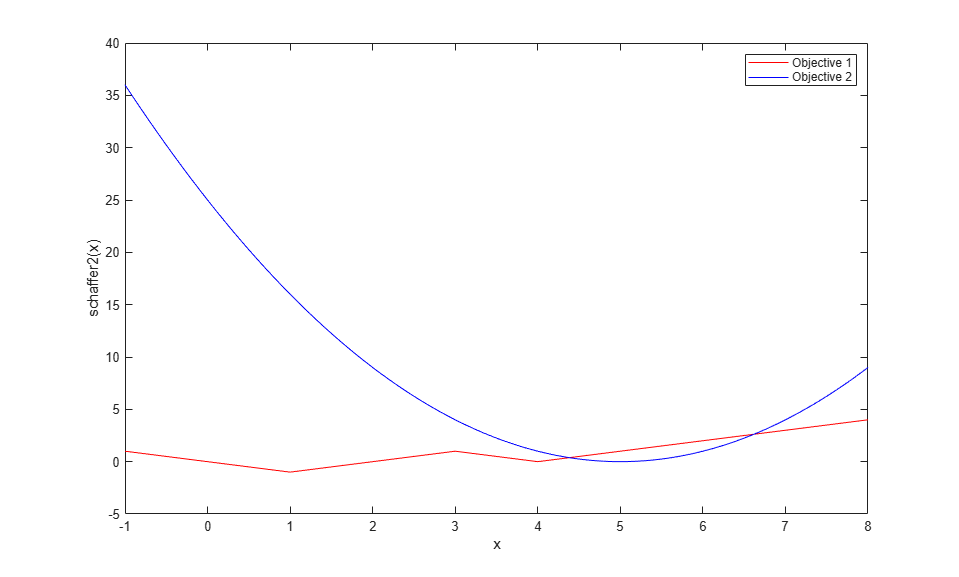The two objective functions compete for `x` in the ranges `[1,3`] and `[4,5]`. But, the Pareto-optimal front consists of only two disconnected regions, corresponding to the `x` in the ranges `[1,2]` and `[4,5]`. There are disconnected regions because the region `[2,3]` is inferior to `[4,5]`. In that range, objective `1` has the same values, but objective `2` is smaller.

Set bounds to keep population members in the range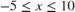.

```lb = -5; ub = 10; ```

Set options to view the Pareto front as `gamultiobj` runs.

```options = optimoptions('gamultiobj','PlotFcn',@gaplotpareto); ```

Call `gamultiobj`.

```rng default % For reproducibility [x,fval,exitflag,output] = gamultiobj(@schaffer2,1,[],[],[],[],lb,ub,options); ```
```Optimization terminated: maximum number of generations exceeded. ```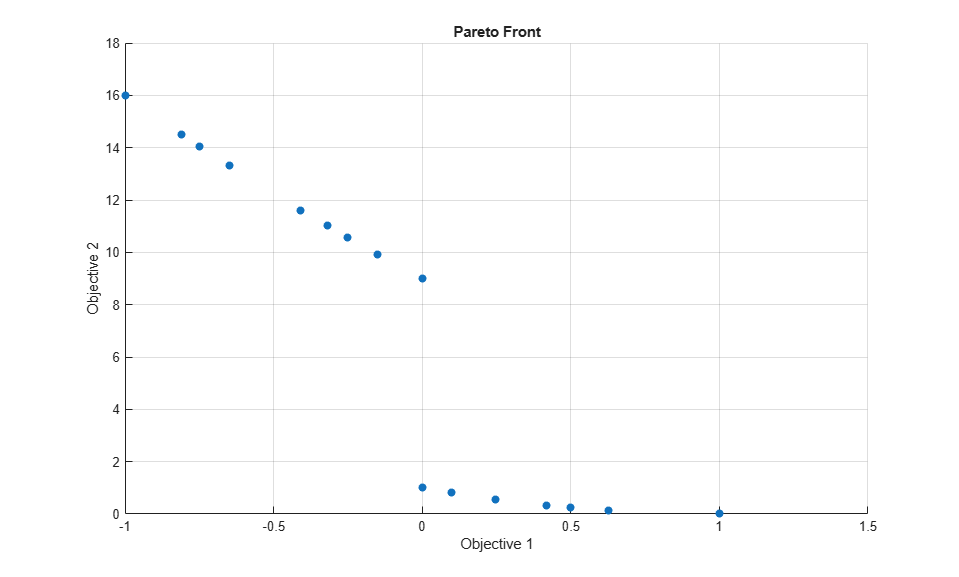Create a two-objective function in two problem variables.

```rng default % For reproducibility M = diag([-1 -1]) + randn(2)/4; % Two problem variables fun = @(x)[(x').^2 / 30 + M*x']; % Two objectives```

Specify that the second variable must be an integer.

`intcon = 2;`

Specify problem bounds, the `gaplotpareto` plot function, and a population size of 100.

```lb = [0 0]; ub = [100 50]; options = optimoptions("gamultiobj","PlotFcn","gaplotpareto",... "PopulationSize",100);```

Find the Pareto set for the problem.

```nvars = 2; A = []; b = []; Aeq = []; beq = []; nonlcon = []; [x,fval] = gamultiobj(fun,nvars,A,b,Aeq,beq,lb,ub,nonlcon,intcon,options);```
```Optimization terminated: average change in the spread of Pareto solutions less than options.FunctionTolerance. ```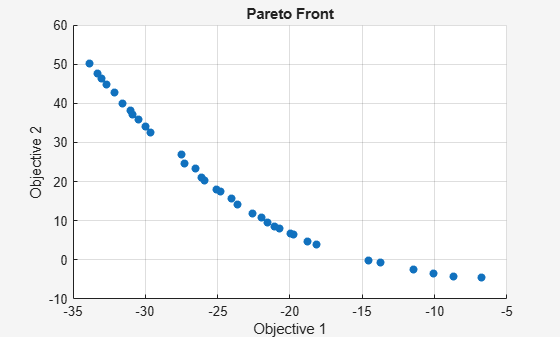List ten of the solutions, and notice that the second variable is integer-valued.

`x(1:10,:)`
```ans = 10×2 8.3393 28.0000 12.9927 49.0000 7.1611 27.0000 7.0210 18.0000 0.0004 12.0000 9.0989 44.0000 9.3974 29.0000 0.5537 17.0000 6.4010 17.0000 7.0531 31.0000 ```

Run a simple multiobjective problem and obtain all available outputs.

Set the random number generator for reproducibility.

```rng default ```

Set the fitness functions to `kur_multiobjective`, a function that has three control variables and returns two fitness function values.

```fitnessfcn = @kur_multiobjective; nvars = 3; ```

The `kur_multiobjective` function has the following code.

```function y = kur_multiobjective(x) %KUR_MULTIOBJECTIVE Objective function for a multiobjective problem. % The Pareto-optimal set for this two-objective problem is nonconvex as % well as disconnected. The function KUR_MULTIOBJECTIVE computes two % objectives and returns a vector y of size 2-by-1. % % Reference: Kalyanmoy Deb, "Multi-Objective Optimization using % Evolutionary Algorithms", John Wiley & Sons ISBN 047187339 % Copyright 2007 The MathWorks, Inc. % Initialize for two objectives y = zeros(2,1); % Compute first objective for i = 1:2 y(1) = y(1) - 10*exp(-0.2*sqrt(x(i)^2 + x(i+1)^2)); end % Compute second objective for i = 1:3 y(2) = y(2) + abs(x(i))^0.8 + 5*sin(x(i)^3); end ```

Set lower and upper bounds on all variables.

```ub = [5 5 5]; lb = -ub; ```

Find the Pareto front and all other outputs for this problem.

```[x,fval,exitflag,output,population,scores] = gamultiobj(fitnessfcn,nvars, ... [],[],[],[],lb,ub); ```
```Optimization terminated: average change in the spread of Pareto solutions less than options.FunctionTolerance. ```

Examine the sizes of some of the returned variables.

```sizex = size(x) sizepopulation = size(population) sizescores = size(scores) ```
```sizex = 18 3 sizepopulation = 50 3 sizescores = 50 2 ```

The returned Pareto front contains 18 points. There are 50 members of the final population. Each `population` row has three dimensions, corresponding to the three decision variables. Each `scores` row has two dimensions, corresponding to the two fitness functions.

## Input Arguments

collapse all

Fitness functions to optimize, specified as a function handle or function name.

`fun` is a function that accepts a real row vector of doubles `x` of length `nvars` and returns a real vector `F(x)` of objective function values. For details on writing `fun`, see Compute Objective Functions.

If you set the `UseVectorized` option to `true`, then `fun` accepts a matrix of size `n`-by-`nvars`, where the matrix represents `n` individuals. `fun` returns a matrix of size `n`-by-`m`, where `m` is the number of objective functions. See Vectorize the Fitness Function.

Example: `@(x)[sin(x),cos(x)]`

Data Types: `char` | `function_handle` | `string`

Number of variables, specified as a positive integer. The solver passes row vectors of length `nvars` to `fun`.

Example: `4`

Data Types: `double`

Linear inequality constraints, specified as a real matrix. `A` is an `M`-by-`nvars` matrix, where `M` is the number of inequalities.

`A` encodes the `M` linear inequalities

`A*x <= b`,

where `x` is the column vector of `nvars` variables `x(:)`, and `b` is a column vector with `M` elements.

For example, give constraints `A = [1,2;3,4;5,6]` and `b = [10;20;30]` to specify these sums:

x1 + 2x2 ≤ 10
3x1 + 4x2 ≤ 20
5x1 + 6x2 ≤ 30.

Example: To set the sum of the x-components to 1 or less, take ```A = ones(1,N)``` and `b = 1`.

Data Types: `double`

Linear inequality constraints, specified as a real vector. `b` is an `M`-element vector related to the `A` matrix. If you pass `b` as a row vector, solvers internally convert `b` to the column vector `b(:)`.

`b` encodes the `M` linear inequalities

`A*x <= b`,

where `x` is the column vector of `nvars` variables `x(:)`, and `A` is a matrix of size `M`-by-`nvars`.

For example, give constraints `A = [1,2;3,4;5,6]` and `b = [10;20;30]` to specify these sums:

x1 + 2x2 ≤ 10
3x1 + 4x2 ≤ 20
5x1 + 6x2 ≤ 30.

Example: To set the sum of the x-components to 1 or less, take ```A = ones(1,N)``` and `b = 1`.

Data Types: `double`

Linear equality constraints, specified as a real matrix. `Aeq` is an `Me`-by-`nvars` matrix, where `Me` is the number of equalities.

`Aeq` encodes the `Me` linear equalities

`Aeq*x = beq`,

where `x` is the column vector of `nvars` variables `x(:)`, and `beq` is a column vector with `Me` elements.

For example, give constraints `Aeq = [1,2,3;2,4,1]` and `beq = [10;20]` to specify these sums:

x1 + 2x2 + 3x3 = 10
2x1 + 4x2 + x3 = 20.

Example: To set the sum of the x-components to 1, take ```Aeq = ones(1,N)``` and `beq = 1`.

Data Types: `double`

Linear equality constraints, specified as a real vector. `beq` is an `Me`-element vector related to the `Aeq` matrix. If you pass `beq` as a row vector, solvers internally convert `beq` to the column vector `beq(:)`.

`beq` encodes the `Me` linear equalities

`Aeq*x = beq`,

where `x` is the column vector of `nvars` variables `x(:)`, and `Aeq` is a matrix of size `Meq`-by-`N`.

For example, give constraints `Aeq = [1,2,3;2,4,1]` and `beq = [10;20]` to specify these sums:

x1 + 2x2 + 3x3 = 10
2x1 + 4x2 + x3 = 20.

Example: To set the sum of the x-components to 1, take ```Aeq = ones(1,N)``` and `beq = 1`.

Data Types: `double`

Lower bounds, specified as a real vector or real array. If `numel(lb) = nvars`, then `lb` specifies that `x(i) >= lb(i)` for all `i`.

If `numel(lb) < nvars`, then `lb` specifies that `x(i) >= lb(i)` for `1 <= i <= numel(lb)`.

In this case, solvers issue a warning.

Example: To specify all x-components as positive, set ```lb = zeros(nvars,1)```.

Data Types: `double`

Upper bounds, specified as a real vector or real array. If `numel(ub) = nvars`, then `ub` specifies that `x(i) <= ub(i)` for all `i`.

If `numel(ub) < nvars`, then `ub` specifies that `x(i) <= ub(i)` for `1 <= i <= numel(ub)`.

In this case, solvers issue a warning.

Example: To specify all x-components as less than one, set ```ub = ones(nvars,1)```.

Data Types: `double`

Nonlinear constraints, specified as a function handle or function name. `nonlcon` is a function that accepts a row vector `x` and returns two row vectors, `c(x)` and `ceq(x)`.

• `c(x)` is the row vector of nonlinear inequality constraints at `x`. The `gamultiobj` function attempts to satisfy `c(x) <= 0` for all entries of `c`.

• `ceq(x)` is the row vector nonlinear equality constraints at `x`. The `gamultiobj` function attempts to satisfy `ceq(x) = 0` for all entries of `ceq`.

If you set the `UseVectorized` option to `true`, then `nonlcon` accepts a matrix of size `n`-by-`nvars`, where the matrix represents `n` individuals. `nonlcon` returns a matrix of size `n`-by-`mc` in the first argument, where `mc` is the number of nonlinear inequality constraints. `nonlcon` returns a matrix of size `n`-by-`mceq` in the second argument, where `mceq` is the number of nonlinear equality constraints. See Vectorize the Fitness Function.

For example, `x = gamultiobj(@myfun,nvars,A,b,Aeq,beq,lb,ub,@mycon)`, where `mycon` is a MATLAB® function such as the following:

```function [c,ceq] = mycon(x) c = ... % Compute nonlinear inequalities at x. ceq = ... % Compute nonlinear equalities at x.```

Data Types: `char` | `function_handle` | `string`

Optimization options, specified as the output of `optimoptions` or a structure. See option details in Genetic Algorithm Options.

`optimoptions` hides the options listed in italics. See Options that optimoptions Hides.

• Values in `{}` denote the default value.

• `{}*` represents the default when there are linear constraints, and for `MutationFcn` also when there are bounds.

• I* indicates that the solver handles options for integer constraints differently.

• NM indicates that the option does not apply to `gamultiobj`.

Options for `ga` and `gamultiobj`

OptionDescriptionValues
`ConstraintTolerance`

Determines the feasibility with respect to nonlinear constraints. Also, `max(sqrt(eps),ConstraintTolerance)` determines feasibility with respect to linear constraints.

For an options structure, use `TolCon`.

Positive scalar | `{1e-3}`

`CreationFcn`

Function that creates the initial population. Specify as a name of a built-in creation function or a function handle. See Population Options.

`{'gacreationuniform'}` | `{'gacreationlinearfeasible'}*` | `'gacreationnonlinearfeasible'` | `{'gacreationuniformint'}`I* for `ga` | `{'gacreationsobol'}`I* for `gamultiobj` | Custom creation function

`CrossoverFcn`

Function that the algorithm uses to create crossover children. Specify as a name of a built-in crossover function or a function handle. See Crossover Options.

`{'crossoverscattered'}` for `ga`, `{'crossoverintermediate'}*` for `gamultiobj` | `{'crossoverlaplace'}`I* | `'crossoverheuristic'` | `'crossoversinglepoint'` | `'crossovertwopoint'` | `'crossoverarithmetic'` | Custom crossover function

`CrossoverFraction`

The fraction of the population at the next generation, not including elite children, that the crossover function creates.

Positive scalar | `{0.8}`

`Display`

Level of display.

`'off'` | `'iter'` | `'diagnose'` | `{'final'}`

`DistanceMeasureFcn`

Function that computes the distance measure of individuals. Specify as a name of a built-in distance measure function or a function handle. The value applies to the decision variable or design space (genotype) or to function space (phenotype). The default `'distancecrowding'` is in function space (phenotype). For `gamultiobj` only. See Multiobjective Options.

For an options structure, use a function handle, not a name.

`{'distancecrowding'}` means the same as `{@distancecrowding,'phenotype'}` | `{@distancecrowding,'genotype'}` | Custom distance function

`EliteCount`

NM Positive integer specifying how many individuals in the current generation are guaranteed to survive to the next generation. Not used in `gamultiobj`.

Positive integer | `{ceil(0.05*PopulationSize)}` | ```{0.05*(default PopulationSize)}``` for mixed-integer problems

`FitnessLimit`

NM If the fitness function attains the value of `FitnessLimit`, the algorithm halts.

Scalar | `{-Inf}`

`FitnessScalingFcn`

Function that scales the values of the fitness function. Specify as a name of a built-in scaling function or a function handle. Option unavailable for `gamultiobj`.

`{'fitscalingrank'}` | `'fitscalingshiftlinear'` | `'fitscalingprop'` | `'fitscalingtop'` | Custom fitness scaling function

`FunctionTolerance`

The algorithm stops if the average relative change in the best fitness function value over `MaxStallGenerations` generations is less than or equal to `FunctionTolerance`. If `StallTest` is `'geometricWeighted'`, then the algorithm stops if the weighted average relative change is less than or equal to `FunctionTolerance`.

For `gamultiobj`, the algorithm stops when the geometric average of the relative change in value of the spread over `options.MaxStallGenerations` generations is less than `options.FunctionTolerance`, and the final spread is less than the mean spread over the past `options.MaxStallGenerations` generations. See gamultiobj Algorithm.

For an options structure, use `TolFun`.

Positive scalar | `{1e-6}` for `ga`, `{1e-4}` for `gamultiobj`

`HybridFcn`

I* Function that continues the optimization after `ga` terminates. Specify as a name or a function handle.

Alternatively, a cell array specifying the hybrid function and its options. See ga Hybrid Function.

For `gamultiobj`, the only hybrid function is `@fgoalattain`. See gamultiobj Hybrid Function.

When the problem has integer constraints, you cannot use a hybrid function.

Function name or handle | ```'fminsearch' | 'patternsearch' | 'fminunc' | 'fmincon' | {[]}```

or

1-by-2 cell array | `{@solver, hybridoptions}`, where ```solver = fminsearch```, `patternsearch`, `fminunc`, or `fmincon` `{[]}`

InitialPenalty

NM I* Initial value of the penalty parameter

Positive scalar | `{10}`

`InitialPopulationMatrix`

Initial population used to seed the genetic algorithm. Has up to `PopulationSize` rows and `N` columns, where `N` is the number of variables. You can pass a partial population, meaning one with fewer than `PopulationSize` rows. In that case, the genetic algorithm uses `CreationFcn` to generate the remaining population members. See Population Options.

For an options structure, use `InitialPopulation`.

Matrix | `{[]}`

`InitialPopulationRange`

Matrix or vector specifying the range of the individuals in the initial population. Applies to `gacreationuniform` creation function. `ga` shifts and scales the default initial range to match any finite bounds.

For an options structure, use `PopInitRange`.

Matrix or vector | `{[-10;10]}` for unbounded components, `{[-1e4+1;1e4+1]}` for unbounded components of integer-constrained problems, `{[lb;ub]}` for bounded components, with the default range modified to match one-sided bounds

`InitialScoresMatrix`

Initial scores used to determine fitness. Has up to `PopulationSize` rows and `Nf` columns, where `Nf` is the number of fitness functions (`1` for `ga`, greater than `1` for `gamultiobj`). You can pass a partial scores matrix, meaning one with fewer than `PopulationSize` rows. In that case, the solver fills in the scores when it evaluates the fitness functions.

For an options structure, use `InitialScores`.

Column vector for single objective | matrix for multiobjective | `{[]}`

`MaxGenerations`

Maximum number of iterations before the algorithm halts.

For an options structure, use `Generations`.

Positive integer |`{100*numberOfVariables}` for `ga`, `{200*numberOfVariables}` for `gamultiobj`

`MaxStallGenerations`

The algorithm stops if the average relative change in the best fitness function value over `MaxStallGenerations` generations is less than or equal to `FunctionTolerance`. If `StallTest` is `'geometricWeighted'`, then the algorithm stops if the weighted average relative change is less than or equal to `FunctionTolerance`.

For `gamultiobj`, the algorithm stops when the geometric average of the relative change in value of the spread over `options.MaxStallGenerations` generations is less than `options.FunctionTolerance`, and the final spread is less than the mean spread over the past `options.MaxStallGenerations` generations. See gamultiobj Algorithm.

For an options structure, use `StallGenLimit`.

Positive integer | `{50}` for `ga`, `{100}` for `gamultiobj`

`MaxStallTime`

NM The algorithm stops if there is no improvement in the objective function for `MaxStallTime` seconds, as measured by `tic` and `toc`.

For an options structure, use `StallTimeLimit`.

Positive scalar `| {Inf}`

`MaxTime`

The algorithm stops after running for `MaxTime` seconds, as measured by `tic` and `toc`. This limit is enforced after each iteration, so `ga` can exceed the limit when an iteration takes substantial time.

For an options structure, use `TimeLimit`.

Positive scalar | `{Inf}`

MigrationDirection

Direction of migration. See Migration Options.

`'both'` | `{'forward'}`

MigrationFraction

Scalar from 0 through 1 specifying the fraction of individuals in each subpopulation that migrates to a different subpopulation. See Migration Options.

Scalar | `{0.2}`

MigrationInterval

Positive integer specifying the number of generations that take place between migrations of individuals between subpopulations. See Migration Options.

Positive integer | `{20}`

`MutationFcn`

Function that produces mutation children. Specify as a name of a built-in mutation function or a function handle. See Mutation Options.

`{'mutationgaussian'}` for `ga` without constraints | `{'mutationadaptfeasible'}*` for `gamultiobj` and for `ga` with constraints | `{'mutationpower'}`I* | `'mutationpositivebasis'` | `'mutationuniform'` | Custom mutation function

`NonlinearConstraintAlgorithm`

Nonlinear constraint algorithm. See Nonlinear Constraint Solver Algorithms for Genetic Algorithm. Option unchangeable for `gamultiobj`.

For an options structure, use `NonlinConAlgorithm`.

`{'auglag'}` for `ga`, `{'penalty'}` for `gamultiobj`

`OutputFcn`

Functions that `ga` calls at each iteration. Specify as a function handle or a cell array of function handles. See Output Function Options.

For an options structure, use `OutputFcns`.

Function handle or cell array of function handles | `{[]}`

`ParetoFraction`

Scalar from 0 through 1 specifying the fraction of individuals to keep on the first Pareto front while the solver selects individuals from higher fronts, for `gamultiobj` only. See Multiobjective Options.

Scalar | `{0.35}`

PenaltyFactor

NM I* Penalty update parameter.

Positive scalar | `{100}`

`PlotFcn`

Function that plots data computed by the algorithm. Specify as a name of a built-in plot function, a function handle, or a cell array of built-in names or function handles. See Plot Options.

For an options structure, use `PlotFcns`.

`ga` or `gamultiobj`: ```{[]} | 'gaplotdistance' | 'gaplotgenealogy' | 'gaplotselection' | 'gaplotscorediversity' |'gaplotscores' | 'gaplotstopping' | 'gaplotmaxconstr' | ```Custom plot function

`ga` only: ```'gaplotbestf' | 'gaplotbestindiv' | 'gaplotexpectation' | 'gaplotrange'```

`gamultiobj` only: ```'gaplotpareto' | 'gaplotparetodistance' | 'gaplotrankhist' | 'gaplotspread'```

PlotInterval

Positive integer specifying the number of generations between consecutive calls to the plot functions.

Positive integer | `{1}`

`PopulationSize`

Size of the population.

Positive integer | `{50}` when ```numberOfVariables <= 5```, `{200}` otherwise | `{min(max(10*nvars,40),100)}` for mixed-integer problems

`PopulationType`

Data type of the population. Must be `'doubleVector'` for mixed-integer problems.

`'bitstring'` | `'custom'` | `{'doubleVector'}`

`ga` ignores all constraints when `PopulationType` is set to `'bitString'` or `'custom'`. See Population Options.

`SelectionFcn`

Function that selects parents of crossover and mutation children. Specify as a name of a built-in selection function or a function handle.

`gamultiobj` uses only `'selectiontournament'`.

`{'selectionstochunif'}` for `ga`, `{'selectiontournament'}` for `gamultiobj` | `'selectionremainder'` | `'selectionuniform'` | `'selectionroulette'` | Custom selection function

StallTest

NM Stopping test type.

`'geometricWeighted'` | `{'averageChange'}`

`UseParallel`

Compute fitness and nonlinear constraint functions in parallel. See Vectorize and Parallel Options (User Function Evaluation) and How to Use Parallel Processing in Global Optimization Toolbox.

`true` | `{false}`

`UseVectorized`

Specifies whether functions are vectorized. See Vectorize and Parallel Options (User Function Evaluation) and Vectorize the Fitness Function.

For an options structure, use `Vectorized` with the values `'on'` or `'off'`.

`true` | `{false}`

Example: `optimoptions('gamultiobj','PlotFcn',@gaplotpareto)`

Integer variables, specified as a vector of positive integers taking values from `1` to `nvars`. Each value in `intcon` represents an `x` component that is integer-valued.

Note

When `intcon` is nonempty, `nonlcon` must return empty for `ceq`.

Example: To specify that the even entries in `x` are integer-valued, set `intcon` to `2:2:nvars`

Data Types: `double`

Problem description, specified as a structure containing these fields.

 `fitnessfcn` Fitness functions `nvars` Number of design variables `Aineq` `A` matrix for linear inequality constraints `Bineq` `b` vector for linear inequality constraints `Aeq` `Aeq` matrix for linear equality constraints `Beq` `beq` vector for linear equality constraints `lb` Lower bound on `x` `ub` Upper bound on `x` `nonlcon` Nonlinear constraint functions `intcon` Indices of integer variables `rngstate` Field to reset the state of the random number generator `solver` `'gamultiobj'` `options` Options created using `optimoptions` or an options structure

You must specify the fields `fitnessfcn`, `nvars`, and `options`. The remainder are optional for `gamultiobj`.

Data Types: `struct`

## Output Arguments

collapse all

Pareto points, returned as an `m`-by-`nvars` array, where `m` is the number of points on the Pareto front. Each row of `x` represents one point on the Pareto front.

Function values on the Pareto front, returned as an `m`-by-`nf` array. `m` is the number of points on the Pareto front, and `nf` is the number of fitness functions. Each row of `fval` represents the function values at one Pareto point in `x`.

Reason `gamultiobj` stopped, returned as an integer.

exitflag ValueStopping Condition
`1`

Geometric average of the relative change in value of the spread over `options.MaxStallGenerations` generations is less than `options.FunctionTolerance`, and the final spread is less than the mean spread over the past `options.MaxStallGenerations` generations

`0`

Maximum number of generations exceeded

`-1`

Optimization terminated by an output function or plot function

`-2`

No feasible point found

`-5`

Time limit exceeded

Information about the optimization process, returned as a structure with these fields.

output FieldMeaning
`problemtype`

Type of problem:

• `'unconstrained'` — No constraints

• `'boundconstraints'` — Only bound constraints

• `'linearconstraints'` — Linear constraints, with or without bound constraints

• `'nonlinearconstr'` — Nonlinear constraints, with or without other types of constraints

`rngstate`

State of the MATLAB random number generator, just before the algorithm started. You can use the values in `rngstate` to reproduce the output of `gamultiobj`. See Reproduce Results.

`generations`Total number of generations, excluding `HybridFcn` iterations.
`funccount`Total number of function evaluations.
`message``gamultiobj` exit message.
`averagedistance`Average “distance,” which by default is the standard deviation of the norm of the difference between Pareto front members and their mean.
`spread`Combination of the “distance,” and a measure of the movement of the points on the Pareto front between the final two iterations.
`maxconstraint`Maximum constraint violation at the final Pareto set.

Final population, returned as an `n`-by-`nvars` array, where `n` is the number of members of the population.

Scores of the final population, returned as an `n`-by-`nf` array. `n` is the number of members of the population, and `nf` is the number of fitness functions.

When there are nonlinear constraints, `gamultiobj` sets the `scores` of infeasible population members to `Inf`.

collapse all

### Pareto Front

A Pareto front is a set of points in parameter space (the space of decision variables) that have noninferior fitness function values.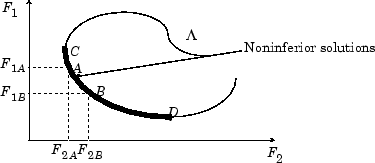In other words, for each point on the Pareto front, you can improve one fitness function only by degrading another. For details, see What Is Multiobjective Optimization?

As in Local vs. Global Optima, it is possible for a Pareto front to be local, but not global. “Local” means that the Pareto points can be noninferior compared to nearby points, but points farther away in parameter space could have lower function values in every component.

## Algorithms

`gamultiobj` uses a controlled, elitist genetic algorithm (a variant of NSGA-II ). An elitist GA always favors individuals with better fitness value (rank). A controlled elitist GA also favors individuals that can help increase the diversity of the population even if they have a lower fitness value. It is important to maintain the diversity of population for convergence to an optimal Pareto front. Diversity is maintained by controlling the elite members of the population as the algorithm progresses. Two options, `ParetoFraction` and `DistanceMeasureFcn`, control the elitism. `ParetoFraction` limits the number of individuals on the Pareto front (elite members). The distance function, selected by `DistanceMeasureFcn`, helps to maintain diversity on a front by favoring individuals that are relatively far away on the front. The algorithm stops if the spread, a measure of the movement of the Pareto front, is small. For details, see gamultiobj Algorithm.

## Alternative Functionality

### App

The Optimize Live Editor task provides a visual interface for `gamultiobj`.

 Deb, Kalyanmoy. Multi-Objective Optimization Using Evolutionary Algorithms. Chichester, England: John Wiley & Sons, 2001.